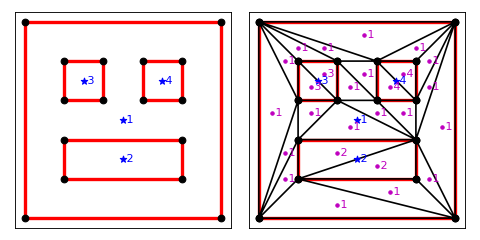# Examples¶

Let us triangulate a simple square

```import matplotlib.pyplot as plt
import numpy as np

import triangle as tr

A = dict(vertices=np.array(((0, 0), (1, 0), (1, 1), (0, 1))))
B = tr.triangulate(A)
tr.compare(plt, A, B)
plt.show()
```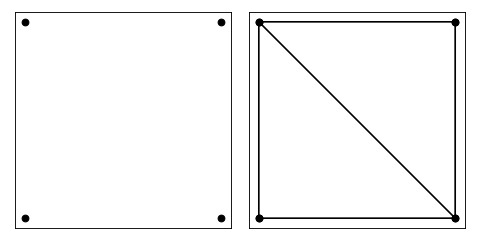In order to set maximum area of the triangles, we set the maxarea keyword

```import matplotlib.pyplot as plt
import numpy as np

import triangle as tr

A = dict(vertices=np.array(((0, 0), (1, 0), (1, 1), (0, 1))))
B = tr.triangulate(A, 'qa0.1')
tr.compare(plt, A, B)
plt.show()
```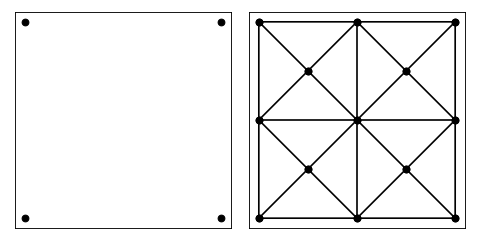If we want to decrease the area even further

```import matplotlib.pyplot as plt
import numpy as np

import triangle as tr

A = dict(vertices=np.array(((0, 0), (1, 0), (1, 1), (0, 1))))
B = tr.triangulate(A, 'qa0.01')
tr.compare(plt, A, B)
plt.show()
```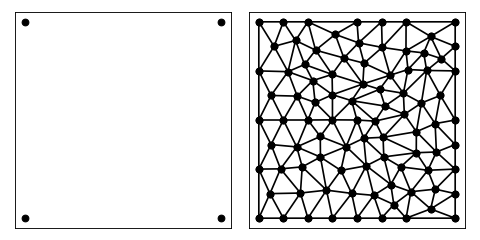To do the same with a circle

```import matplotlib.pyplot as plt
import numpy as np

import triangle as tr

N = 32
theta = np.linspace(0, 2 * np.pi, N, endpoint=False)
pts = np.stack([np.cos(theta), np.sin(theta)], axis=1)
A = dict(vertices=pts)
B = tr.triangulate(A, 'q')
tr.compare(plt, A, B)
plt.show()
```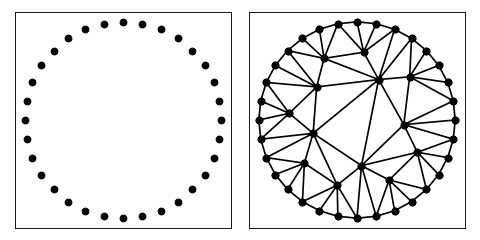```import matplotlib.pyplot as plt
import numpy as np

import triangle as tr

N = 32
theta = np.linspace(0, 2 * np.pi, N, endpoint=False)
pts = np.stack([np.cos(theta), np.sin(theta)], axis=1)
A = dict(vertices=pts)
B = tr.triangulate(A, 'qa0.05')
tr.compare(plt, A, B)
plt.show()
```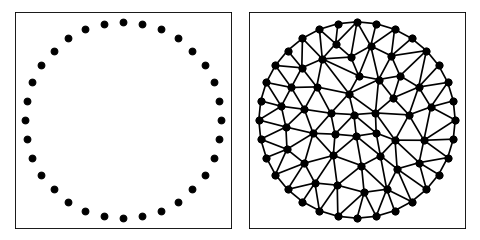To add a hole in the middle of the circle

```import matplotlib.pyplot as plt
import numpy as np

import triangle as tr

def circle(N, R):
i = np.arange(N)
theta = i * 2 * np.pi / N
pts = np.stack([np.cos(theta), np.sin(theta)], axis=1) * R
seg = np.stack([i, i + 1], axis=1) % N
return pts, seg

pts0, seg0 = circle(30, 1.4)
pts1, seg1 = circle(16, 0.6)
pts = np.vstack([pts0, pts1])
seg = np.vstack([seg0, seg1 + seg0.shape])

A = dict(vertices=pts, segments=seg, holes=[[0, 0]])
B = tr.triangulate(A, 'qpa0.05')
tr.compare(plt, A, B)
plt.show()
```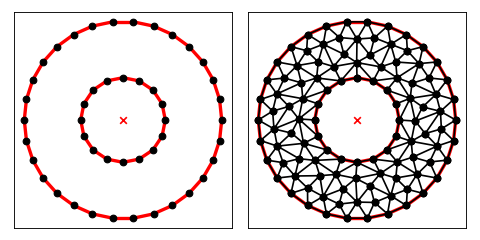To triangulate with region attributes

```import matplotlib.pyplot as plt
import numpy as np

import triangle as tr

# arrays to fill in with input
vertices = []
segments = []
regions = []

# make a box with given dims and place given attribute at its center
def make_box(x, y, w, h, attribute):

i = len(vertices)

vertices.extend([[x,   y],
[x+w, y],
[x+w, y+h],
[x,   y+h]])

segments.extend([(i+0, i+1),
(i+1, i+2),
(i+2, i+3),
(i+3, i+0)])

regions.append([x+0.5*w, y+0.5*h, attribute, 0])

# generate some input
make_box(0, 0, 5, 5, 1)
make_box(1, 1, 3, 1, 2)
make_box(1, 3, 1, 1, 3)
make_box(3, 3, 1, 1, 4)

A = dict(vertices=vertices, segments=segments, regions=regions)
B = tr.triangulate(A, 'pA')

tr.compare(plt, A, B)
plt.show()
```